Wald identity

An identity in sequential analysis which states that the mathematical expectation of the sumof a random numberof independent, identically-distributed random variables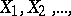is equal to the product of the mathematical expectations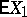and:A sufficient condition for the Wald identity to be valid is that the mathematical expectations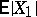and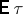in fact exist, and for the random variableto be a Markov time (i.e. for anythe event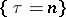is determined by the values of the random variablesor, which is the same thing, the eventbelongs to the-algebra generated by the random variables). Wald's identity is a particular case of a fundamental theorem in sequential analysis stating that(*)
for all complexfor which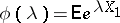exists and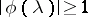. It was established by A. Wald .